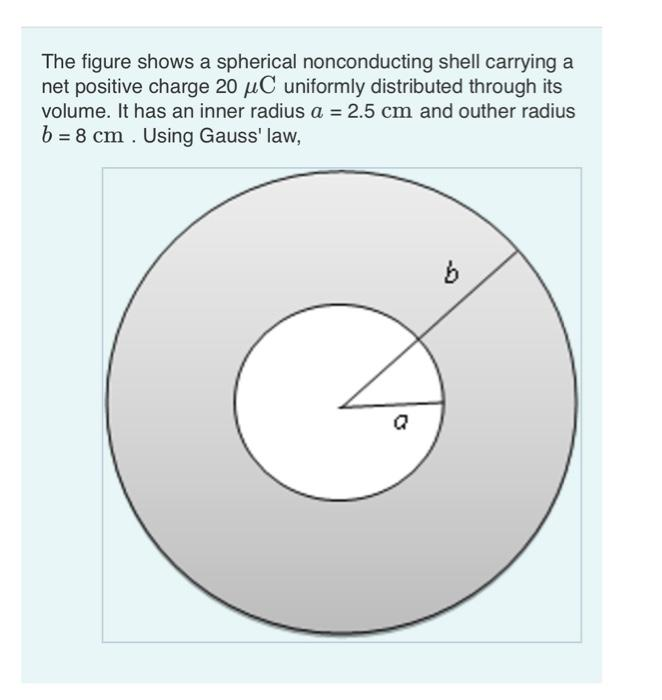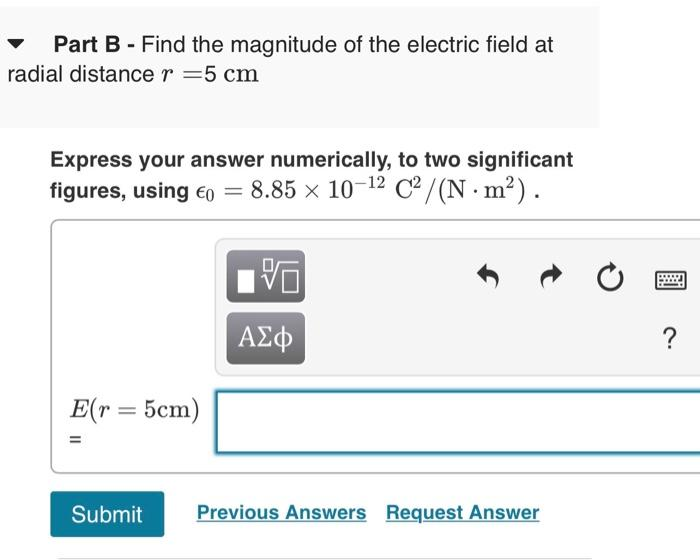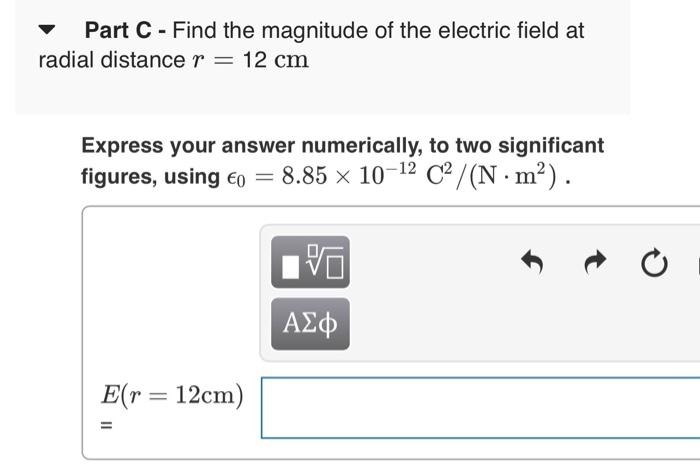# Question The figure shows a spherical nonconducting shell carrying a net positive charge $$20 \mu \mathrm{C}$$ uniformly distributed through its volume. It has an inner radius $$a=2.5 \mathrm{~cm}$$ and outher radius $$b=8 \mathrm{~cm}$$. Using Gauss' law, Part B - Find the magnitude of the electric field at radial distance $$r=5 \mathrm{~cm}$$ Express your answer numerically, to two significant figures, using $$\epsilon_{0}=8.85 \times 10^{-12} \mathrm{C}^{2} /\left(\mathrm{N} \cdot \mathrm{m}^{2}\right)$$. $E(r=5 \mathrm{~cm})$ Part C - Find the magnitude of the electric field at radial distance $$r=12 \mathrm{~cm}$$ Express your answer numerically, to two significant figures, using $$\epsilon_{0}=8.85 \times 10^{-12} \mathrm{C}^{2} /\left(\mathrm{N} \cdot \mathrm{m}^{2}\right)$$. $E(r=12 \mathrm{~cm})$Transcribed Image Text: The figure shows a spherical nonconducting shell carrying a net positive charge $$20 \mu \mathrm{C}$$ uniformly distributed through its volume. It has an inner radius $$a=2.5 \mathrm{~cm}$$ and outher radius $$b=8 \mathrm{~cm}$$. Using Gauss' law, Part B - Find the magnitude of the electric field at radial distance $$r=5 \mathrm{~cm}$$ Express your answer numerically, to two significant figures, using $$\epsilon_{0}=8.85 \times 10^{-12} \mathrm{C}^{2} /\left(\mathrm{N} \cdot \mathrm{m}^{2}\right)$$. $E(r=5 \mathrm{~cm})$ Part C - Find the magnitude of the electric field at radial distance $$r=12 \mathrm{~cm}$$ Express your answer numerically, to two significant figures, using $$\epsilon_{0}=8.85 \times 10^{-12} \mathrm{C}^{2} /\left(\mathrm{N} \cdot \mathrm{m}^{2}\right)$$. $E(r=12 \mathrm{~cm})$
Transcribed Image Text: The figure shows a spherical nonconducting shell carrying a net positive charge $$20 \mu \mathrm{C}$$ uniformly distributed through its volume. It has an inner radius $$a=2.5 \mathrm{~cm}$$ and outher radius $$b=8 \mathrm{~cm}$$. Using Gauss' law, Part B - Find the magnitude of the electric field at radial distance $$r=5 \mathrm{~cm}$$ Express your answer numerically, to two significant figures, using $$\epsilon_{0}=8.85 \times 10^{-12} \mathrm{C}^{2} /\left(\mathrm{N} \cdot \mathrm{m}^{2}\right)$$. $E(r=5 \mathrm{~cm})$ Part C - Find the magnitude of the electric field at radial distance $$r=12 \mathrm{~cm}$$ Express your answer numerically, to two significant figures, using $$\epsilon_{0}=8.85 \times 10^{-12} \mathrm{C}^{2} /\left(\mathrm{N} \cdot \mathrm{m}^{2}\right)$$. $E(r=12 \mathrm{~cm})$&#12304;General guidance&#12305;The answer provided below has been developed in a clear step by step manner.Step1/2Given data are\begin{align*} \mathrm{{Q}} &= \mathrm{{20}\mu{C}} \end{align*}Inner radius \begin{align*} \mathrm{{a}} &= \mathrm{{2.5}{c}{m}} \end{align*}Outer radius\begin{align*} \mathrm{{b}} &= \mathrm{{8}{c}{m}} \end{align*}Total charge in the volume is calculated as{:[a=2.5cm],[b=8cm],[Q=20 muC]:}a) r=5cmb r=12cm{:[" charge density "=(" charge ")/(" total vilures ")=(Q)/((4)/(3)pi(b^(3)-a^(3)))=(20 xx10^(-6))/((4)/(3)pi(8^(3)-2.5^(3))xx(10^(-2))],[rho=(3xx20 xx10^(-6))/(4xx3.14 xx(512-15.625)xx10^(-6))=9.624 xx10^(-3)C]:}Using Gam's law ointEds=(1)/(epsi_(0))int rho*dt.CS Scanned with CamScannerExplanation:Please refer to solution in this step.Step2/2The field at any point can be calculated by Gausses law\( \ ... See the full answer